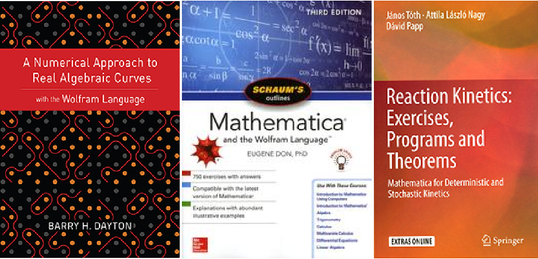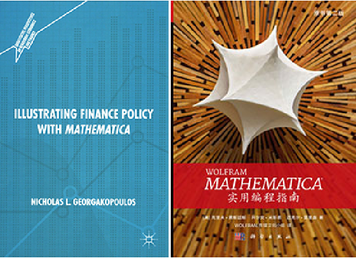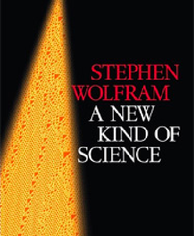Browse by Topic
Related Topics

# New Books, New Possibilities: The Latest Additions to the Wolfram Bookshelf

Check out these fresh picks from authors utilizing the Wolfram Language! Covering a range of topics from algebraic curves to reaction kinetics to finance policy, these books are excellent additions to the extensive list of publications showing what’s possible with Wolfram technologies.## A Numerical Approach to Real Algebraic Curves with the Wolfram Language

Classical treatments of real algebraic curves rely on rational numbers, making analysis possible only in the theoretical sense. But as author Barry H. Dayton shows in this book, the Wolfram Language’s machine-precision number capabilities enable accurate analysis of extremely complicated curves that often lack rational points. The book is written for those with some understanding of partial derivatives and calculus, as well as a basic knowledge of the Wolfram Language.

A Numerical Approach to Real Algebraic Curves with the Wolfram Language was published through Wolfram Media, the publishing unit of the Wolfram Group, and typeset with our book-publishing template. Dayton is the first Wolfram Media–published author not affiliated with Wolfram Research, with many more such authors to come.

More books from Wolfram Media are in the works, so watch this space! If you’re an author looking to publish your own Wolfram technology–related manuscript, please reach out to Wolfram Media.

## Schaum’s Outline of Mathematica and the Wolfram Language, Third Edition (Schaum’s Outlines)

Author Eugene Don adds this Mathematica-focused textbook to Schaum’s Outlines, a series of supplementary textbooks trusted by over 40 million students since the 1930s. In this book, Don introduces the reader to the Wolfram Language, gives a reference index of essential Mathematica functions and provides 750 exercises (with answers included). Schaum’s Outline of Mathematica and the Wolfram Language can be used as a support text for all major textbooks required for courses using Mathematica.

## Reaction Kinetics: Exercises, Programs and Theorems: Mathematica for Deterministic and Stochastic Kinetics

Fifty years ago, an approach to reaction kinetics based on mathematical models of reaction kinetics (formal reaction kinetics) emerged. In the years since, there’s been an accelerated development in deterministic and stochastic kinetics. Based on recent papers, authors János Tóth, Attila László Nagy and Dávid Papp present the most important concepts and results in reaction kinetics in an effort to make the information gathered accessible to a wider audience. The book is accompanied by the authors’ Mathematica package ReactionKinectics.## Illustrating Finance Policy with Mathematica

Understanding quantitative aspects of finance and policy analysis is important for the education of students in various disciplines. However, those students often have little quantitative background. Nicholas L. Georgakopoulos discusses these topics and explains how to illustrate them using Mathematica’s powerful visual capabilities. This book introduces the reader to Mathematica, shows how to use Mathematica to produce illustrations and emphasizes discussion of finance and policy.

## Hands-on Start to Wolfram Mathematica and Programming with the Wolfram Language (in Chinese)

With their popular book now available in Chinese (as well as English and Japanese), authors Cliff Hastings, Kelvin Mischo and Michael Morrison have expanded their catch-all guide to learning and understanding Mathematica. Updates include new content for 3D printing, graphics capabilities and working with audio, data, dates and linguistic data.

## A New Kind of Science—Now in PaperbackStarting from a collection of simple computer experiments—illustrated in the book by striking computer graphics—Stephen Wolfram shows how their unexpected results force a whole new way of looking at the operation of our universe. Wolfram uses his approach to tackle a remarkable array of fundamental problems in science: from the origin of the Second Law of Thermodynamics to the development of complexity in biology, the computational limitations of mathematics, the possibility of a truly fundamental theory of physics and the interplay between free will and determinism.

 Free shipping Get free ground shipping on A New Kind of Science (paperback) through December 31, 2018. Buy now!